# KSEEB Solutions for Class 9 Maths Chapter 13 Surface Area and Volumes Ex 13.6

## Karnataka Board Class 9 Maths Chapter 13 Surface Area and Volumes Ex 13.6

(Assume π = $$\frac{22}{7}$$ unless stated otherwise)

Question 1.
The circumference of the base of a cylindrical vessel is 132 cm and its height is 25 cm. How many litres of water can it hold ? (1000 cm3 = 1 l)
Solution:
The circumference of the base of cylindrical vessel, C = 132 cm.
height, h = 25 cm
C = 2πr = 132r = 21 cm
∴ Volume of Cylinder, V = πr2h= 34650 cm3
1000 cubic cm. = 1 litre
∴ 34650 cm3 = … ? …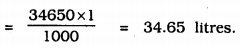Question 2.
The inner diameter of a cylindrical wooden pipe is 24 cm and its outer diameter is 28 cm. The length of the pipe is 35 cm. Find the mass of the pipe, if 1 cm3 of wood has a mass of 0.6 g.
Solution:
Let the inner diameter of a cylindrical vessel be d1 and outer diameter be d2.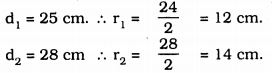height of pipe, h = 35 cm.
∴ volume of pipe, V = $$\pi r_{2}^{2} h-\pi r_{1}^{2} h$$= 110 × 52
∴ V = 5720 cm3
Mass of pipe of 1 ccm is 0.6 gm.
∴ Mass of 5720 cm3. … ? …
= 5720 × 0.6
= 3432 Kg.

Question 3.
A soft drink is available in two packs –
(i) a tin can with a rectangular base of length 5 cm and width 4 cm, having a height of 15 cm and
(ii) a plastic cylinder with circular base of diameter 7 cm and height 10 cm.
Which container has greater capacity and by how much ?
Solution:
Length of rectangular tin, l = 5 cm,
height, h = 15 cm.
∴ Volume of rectangular tin, V = l × b × h
V = 5 × 4 × 15
= 300 cm3
Diameter of plastic cylinder, d = 7 cm,
∴ r = $$\frac{7}{2}$$
Volume of cylinder, v = $$\pi \mathrm{r}^{2} \mathrm{h}$$= 11 × 7 × 5
= 385 cm3.
∴ Plastic cylinder’s volume is great.
= Volume of cylindrical tin – Volume of rectangular tin.
= 385 – 300
= 85 cm3.
∴ Volume of plastic tin is greater than rectangular tin by 85 cm3.

Question 4.
If the lateral surface of a cylinder is 94.2 cm2 and its height is 5 cm, then find
(ii) its volume. (Use π = 3.14)
Solution:
Curved surface area of cylinder = 94.2 cm2
height, h = 5 cm.
r = ?
V = ?
(i) Curved Surface area of cylinder, L.S.A.
A= 2πrh
94.2 = 2 × 3.14 × 5 × r= 3.01 cm.
= 3 cm.
(ii) Volume of cylinder, V = πr2h
= 3.14 × (3)2 × 5
= 3.14 × 9 × 5
= 141.3 cm3.

Question 5.
It costs Rs. 2200 to paint the inner curved surface of a cylindrical vessel 10 m deep. If the cost of painting is at the rate of Rs. 20 per m2, find
(i) inner curved surface area of the vessel.
(iii) capacity of the vessel.
Solution:
(i) Cost of painting for cylindrical vessel = Rs. 2200
Cost of painting is at the rate of Rs. 20 per m2.
∴ Curved Surface area of vessel = $$\frac{2200}{20}$$
= 110 m2

(ii) Depth of vessel, h = 10 m
r = ?
Curved surface area of vessel, V = 2πrh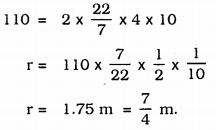(iii) Capacity of the Vessel = Volume of Vessel
V = πr2hV = 96.25 m3.

Question 6.
The capacity of a closed cylindrical vessel of height 1 m is 15.4 litres. How many square metres of metal sheet would be needed to make it ?
Solution:
Volume of a cylindrical vessel = 15.4 litres
1000 c.cm. = 1 litre.height, h = 1 m,
Curved surface area =?
Volume of cylinder, V = πr2h
0. 0154 = $$\frac{22}{7}$$ × r2 × 1r = 0.7 m
Now, curved surface area of cylinder vessel,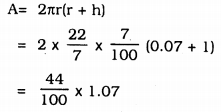= 0.44 × 1.07
A = 0.4708 m2

Question 7.
A lead pencil consists of a cylinder of wood with a solid cylinder of graphite filled in the interior. The diameter of the pencil is 7 mm and the diameter of the graphite is 1 mm. If the length of the pencil is 14 cm, find the volume of the wood and that of the graphite.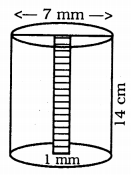Solution:
Diameter of pencil, d = 7 mm = 0.7 m.length of pencil, h = 14 cm
diameter of pencil, d = 1 mm = 0.1 cmVolume of wood in pencil, V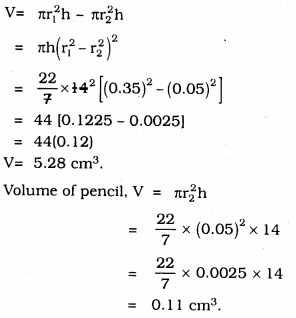Question 8.
A patient in a hospital is given soup daily in a cylindrical bowl of diameter 7 cm. If the bowl is filled with soup to a height of 4 cm, how much soup the hospital has to prepare daily to serve 250 patients?
Solution:
Diameter of pencil, d = 7 cmheight of soup, h = 4 cm
Volume of soup given for 1 patient is 154 cm3
Volume of soup given for 250 patients .. ? ….
= 154 × 250 = 38500 cm3
For 1000 cm3, 1 litre
For 38500 cm3 ….. ? …
= $$\frac{38500}{1000}$$
= 38.5 litres soup.

##### KSEEB Solutions for Class 9 Maths
error: Content is protected !!